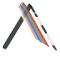Class Central is learner-supported. When you buy through links on our site, we may earn an affiliate commission.

Matrix Methods

Overview

Class Central Tips
Mathematical Matrix Methods lie at the root of most methods of machine learning and data analysis of tabular data. Learn the basics of Matrix Methods, including matrix-matrix multiplication, solving linear equations, orthogonality, and best least squares approximation. Discover the Singular Value Decomposition that plays a fundamental role in dimensionality reduction, Principal Component Analysis, and noise reduction. Optional examples using Python are used to illustrate the concepts and allow the learner to experiment with the algorithms.

Syllabus

• Matrices as Mathematical Objects
• Matrix Multiplication and other Operations
• Systems of Linear Equations
• Linear Least Squares
• Singular Value Decomposition

Daniel Boley

Reviews

3.0 rating, based on 1 reviews

Start your review of Matrix Methods

•Agrover112
1
The following course offers some really good reference materials which helps in studying the mathematical concepts.

Do not skip the reference materials.

The SVD section is the best intutive and mathematical explanation of SVD ever! You will learn how small changes to the SVD matrix would effect the entire outcome !

The instructor does seem bit uninterested at times .

I would suggest Gilbert Strange Lin Alg lecs as a ref . for this.Never Stop Learning!

Get personalized course recommendations, track subjects and courses with reminders, and more.